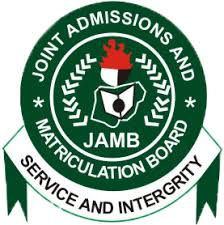HomeJambWaecNecoNabtebNews
Welccome To Examloaded.Com>>>>  Nigeria's No 1 Exam Expo Website, With 7 Years + of Experience Verified by Google #Na We Sure Pass!
Our Only Contact | Call | Text| WhatsApp08162563540

SUBSCRIBE HERE NOW!!# Neco 2019/2020 ssce Mathematics Syllabus/Brochure Questions & Answers

MATHEMATICS OBJ:
1-10 CDAAEABAEC
11-20 AEDDCDCDCC
21-30 CEBDEDCBBC
31-40 CBEEECBDCC
41-50 DBCBCDDBCA
51-60 BCBDCDCCEC

(1a)
Log10(20x-10) – log10(x+3)= log10^5
Log10(20x-10)/x+3) =log10^5
20x-10/x+3 = 5
Cross multiply
20x-10 = 5(x+3)
5(4x-2) = 5(x+3)
4x-x = 3+2
3x= 5
X=3/5

(1b)
Let actual amount be #x
15% of #x = #600
15x/100 =600
x =(100/15) * 600
x = 100 * 40
x = 4,000
Actual amount = 4,000

(2a)
(X^2 Y^-3 Z)^3/4/X^-1 Y^4 Z^5
= (X^2)^3/4/X^-1 * (Y^-3)^3/4/Y^4 * Z^3/4/Z^5
= X^3/2/X^-1 * Y^-9/4/Y^4 * Z^3/4/Z^5
=X^3/2+1 * Y^-9/4-4 * Z^3/4-5
=X^5/2 * Y^-25/4 * Z^-17/4
=X^10/4 * Y^-25/4 * Z^-17/4

=(X^10/Y^25 Z^17)^1/4

(2b)
√2/k + √2 = 1/k – √2
Multiply both sides by (k+√2)(k-√2)
√2(k-√2) = k+√2
√2k-√2 = k+√2
√2k-k = 2+√2
K(√2 -1) = 2+√2
K = 2+√2/√2-1
K = -(2+√2)/1-√2
Rationalizing
K = -(2+√2) * 1+√2/1-√2
K = -(2+√2)(1+√2)/1 – 2
K = (2+√2)(1+√2)
K = 2+2√2 + √2+2
K = 4+3√2

(3)
V = Mg√1 – r²
Square both sides
V² = m²g²(1-r²)
V²/m²g² = 1-r²
r² = 1 – v²/m²g²
r = √1-(v/mg)²
If v = 15, m = 20, and g = 10
r = √1 – (15/20*10)²
r = √1 – (0.075)²
r= √(1.075)(0.925)
r = √0.994375
r = 0.9972

(4ai)
length of Arc of the sector
Titter= 72?, r = 14cm
L= titter / 360 x 2 pie r
==> L= 72/360 x 2 x 22/7 x 14
=44352/2520 = 17.6cm

(ii) perimeter of the sector
Perimeter = titter/360 x 2 pie r + 2r = 17.6 +(2×14) =17.6+28= 45.6cm

(iii) Area of the sector
Area = Titter/360 x pie r? =
72/360 x 22/7 x (14)? = 72 x 22 x 196/2520
Area= 310464/2520 = 123.2cm?

(5a)
Mode = mass with highest frequency = 35kg
Median is the 18th mass
= 40kg.

(5b)
IN A TABULAR FORM

Under Masses(x kg)
30,35,40,45,50,55

Under frequency(f)
5,9,7,6,4,4
Ef = 35

Under X-A
-10, -5, 0, 5, 10, 15

Under F(X-A)
-50, -45, 0, 30, 40, 60
Ef(X – A) = 35

Mean = A + (Ef(X – A)/Ef)
= 40 + 35/35
= 40 + 1
= 41kg

(6)
(a) log2 = 0.3010
Log3 base 10 = 0.4771
(i) Log10 3.6 = Log10 36/10
= log10 36 – log10 base 10
= log10 (9×4) -1
=log10 9+log10 4 – 1
=log10 3² + log10 2² – 1
=2log10 3 + 2log10 2 – 1
= 2(0.4771) +2(0.3010) -1
= 0.9542 + 0.6020 – 1
= 0.5562

(6aii)
Log10 0.9
= log10 9/10 = log10 9-log10 10
= 2log10 3 – 1
= 2(0.4771)-1
= -0.0458
= 1.9542

(6b)
(3√5 – 4√5)(3√5-4√5)/(3√5+4√5)(3√5-4√5)
= 45 – 60 + 80 = 60
45-60+60-80
= 5/35 = 1/7

(7ai)
T3=>a+2d=6(eqi)
T7=>a+6d=30(eqii)
Eqii minus eqi gives
6d-2d=30-6
4d=24
d=24/4
d=6
Common difference=6

(7aii)
Putting d=6 into eqi
a+2(6)=6
a+12=6
a=6-12
a=-6
(7aiii)
10th term T10=a+9d
=-6+9(6)
=-6+54
=48

(7bi)
T3=>ar²=9/2(eqi)
T6=>ar^5=243/16(eqii)
Dividing eqii by eqi
ar^5/ar²=243/16 divided by 9/2
r³=243/16*2/9
r³=27/8
r³=3³/2³
r=3/2
Putting this into eqi
a(3/2)²=9/2
a(9/4)=9/2
a=9/2*4/9
a=4/2=2

(7bii)
Common ratio r=3/2 as above

(8)
x=a+by(eqi)
when y=5 and x=19
19=a+5b(eqii)
when y=10 and x=34
34=a+10b(eqiii)
solving eqii and eqiii
a+10b=34
a+5b=19
=>5b=15
b=15/5=3
putting b=3 in eqii
19=a+5(3)
19=a+15
a=19-15
a=4

(8i)
Putting a=4 and b=3 in eqi
x=4+3y
This is the relationship between xand y

(8ii)
When y=7
x=4+3(7)
x=4+21
x=25

(8b)
3x/x+2 – 5x/3x-1 + 1/5
Find the LCM

3(3x-1)(3x)-3(x+2)(5x)+(+2)3x-1/(x+2)(3x-1)(3)
27x² = 9x – 15x²-30x + 3x²-x+6x-2/3(x+2)(3x-1)

Collect the lime terms
15x² – 34 – 2/3(x+2)(3x-1)

(10a)
Obtuse <BOD + Reflex<BOD = 360degrees (angle at a point)
105 + reflex<BOD = 360degrees
Reflex <BOD= 360 – 105
=255°
Now 2w = reflex<BOD(angle at centre = twice angle at circumference)
2w =255°
W = 255/2 =127.5°

Also 2x = obtuse<BOD(angle at centre = twice angle at circumference)
2x = 105°
X = 105/2 = 52.5°
Now EDF = y(base angles of an isosceles triangle)
BED=X=52.5°(angles in the same segment)
EFD+EDF=BED (sum of interior angles of a triangle equal exterior angle)
Y+y = 52.5°
2y = 52.5°
Y = 52.5°/2
=26.25°

(10b)
Draw the diagram
|TB|/|BR| = TanR
100/|BR| = Tan60°
|BR| = 100/tan60
|BR| = 100√3
|BR| = 100√3 * √3/√3
=100√3/3m OR 57.7m

11a)
x+y/2 =11
x+y= 11*2
x+y= 22 —(1)
x-y= 4 —-(11)
x+y = 22—-(1)

x-y= 4—-(11)
____________
2y = 18
y= 18/2
y=9
Substitute y=9 in equ 1
x+9=22
x=22-9
x=13
x=13, y=9
x+y= 13+9= 22
Sum of the two number

(11b)
(6x + 3) dx
(6x + 3)dx
(6x +3)^6 – (6x + 3)^1
(6 x + 3)^5
(7776x^5 + 243)
38,880x/6 + 243
6480 x^6 + 243x
9(720x^6 + 27x)

(11c)
y = x² + 5x – 3 (x = 2)
y = 2² + 5(2) – 3
y = 4 + 10 – 3
y = 14 – 3
y = 11
Gradient of the curve = 11

(12a)
Pr of Abu to pass = 3/7
Pr of Abu to fail = 1 – 3/7 = 7-3/7 = 4/7

Pr of kuranku to pass = 5/9
Pr of kuranku to fail = 1 – 5/9 = 9 – 5/9 = 4/9

Pr of musa to pass = 12/13
Pr of musa to fail = 1 – 12/13 = 13 – 12/13 = 1/13

Pr of only one of them passing is
=(3/7*4/9*1/13)+(5/9*4/7*1/13)+(12/13*4/7*4/9)
=12/819+ 20/819 + 192/819
=12+20+192/819 = 224/819
= 32/117

(12b)
10Red + 8green + 7blue = 25

(i)
pr of different colour is
Prof(RG)+(RB)+(GB)+(BG)+(BR) +(GR)
=(10/25*8/24)+(10/25*7/24)+(8/25*7/24)+(7/25*8/24)+(7/25*10/24)+(8/25*10/24)
=80/100 + 70/600 + 56/600 + 56/600 + 70/600 + 80/600
= 80+70+56+56+70+80/600
= 412/800 = 103/200

(ii)
pr of atleast one must be
=Pr[RB+BR+GB+BG+BB]
= (10/25*7/24)+(7/25*10/24)+(8/25*7/24)+ (7/25*8/24) + (7/25*7/24)
=70/600+70/600+56/600+56/600+49/600
=70+70+56+56+49
/600
=301/600